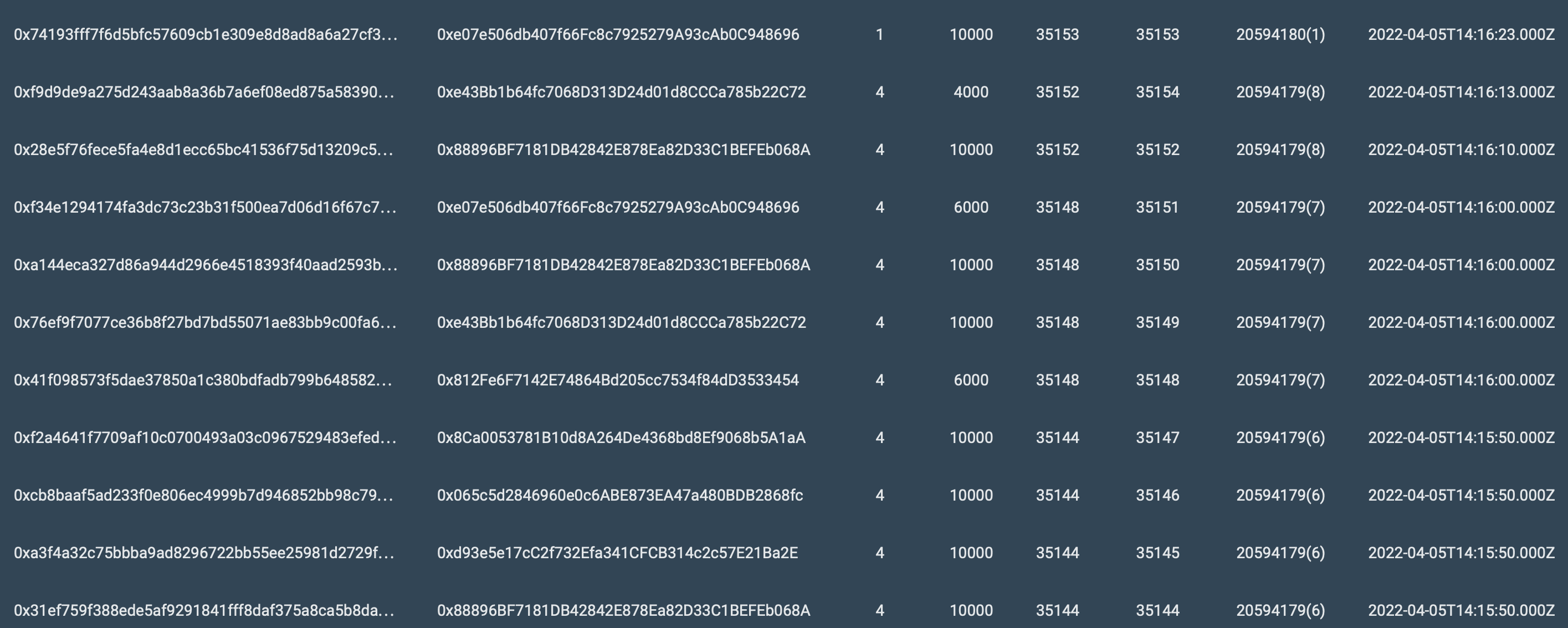# Testnet 3 Test 9

For the test, we used Amazon EC2 T3 Instances. With the following server characteristics:

• Name: `t3a.small`
• vCPUs: `2`
• Memory (GiB): `2.0`
• Baseline Performance/vCPU: `20%`
• CPU Credits earned/hr: `24`
• Network burst bandwidth (Gbps): `5`
• EBS burst bandwidth (Mbps): `Up to 2,085`

Algorithm of actions during testing:

1. Stop creating blocks in the tested network.
2. Generates ~96,000 transactions.
3. Start 64 nodes.
4. Fix the time in which all these transactions were added and accepted into blocks, i.e. executed.

Let’s analyze the line from the test results below:

Block number: 35144
Number of transactions: 10000
Age(slot): 20594179(6)

Block number: 35145
Number of transactions: 10000
Age(slot): 20594179(6)

Block number: 35146
Number of transactions: 10000
Age(slot): 20594179(6)

Block number: 35147
Number of transactions: 10000
Age(slot): 20594179(6)

Block number: 35148
Number of transactions: 6000
Age(slot): 20594179(7)

Block number: 35149
Number of transactions: 10000
Age(slot): 20594179(7)

Block number: 35150
Number of transactions: 10000
Age(slot): 20594179(7)

Block number: 35151
Number of transactions: 6000
Age(slot): 20594179(7)

Block number: 35152
Number of transactions: 10000
Age(slot): 20594179(8)

Block number: 35154
Number of transactions: 4000
Age(slot): 20594179(8)

Let’s check how many transactions were added and processed in the two slots.
Take 2 slots = 20 seconds.
20594179(6)-20594179(7)
(10000+10000+10000+10000 + 10000+6000+10000+6000 ) = 72000

72000/20=3600 TPS.

Now let’s check the values for three slots.
If we take the 3rd slot (20594179(8)) (here we have a non-uniform distribution of transactions, since there were ~80000 in total).
(72000+10000+4000) = 96000

96000/30=3200 TPS.